# Coordinate Systems, Transformations and Units

## Introduction

For all media, the canvas describes "the space where the SVG content is rendered." The canvas is infinite for each dimension of the space, but rendering occurs relative to a finite rectangular region of the canvas. This finite rectangular region is called the SVG viewport. For visual media ([CSS2], section 7.3.1), the SVG viewport is the viewing area where the user sees the SVG content.

The size of the SVG viewport (i.e., its width and height) is determined by a negotiation process (see Establishing the size of the initial viewport) between the SVG document fragment and its parent (real or implicit). Once the viewport is established, the SVG user agent must establish the initial viewport coordinate system and the initial user coordinate system (see Initial coordinate system). The viewport coordinate system is also called viewport space and the user coordinate system is also called user space.

A new user space (i.e., a new current coordinate system) can be established at any place within an SVG document fragment by specifying transformations in the form of transformation matrices or simple transformation operations such as rotation, skewing, scaling and translation (see Coordinate system transformations). Establishing new user spaces via coordinate system transformations are fundamental operations to 2D graphics and represent the usual method of controlling the size, position, rotation and skew of graphic objects.

New viewports also can be established. By establishing a new viewport, one can provide a new reference rectangle for "fitting" a graphic into a particular rectangular area. ("Fit" means that a given graphic is transformed in such a way that its bounding box in user space aligns exactly with the edges of a given viewport.)

## The initial viewport

The SVG user agent negotiates with its parent user agent to determine the viewport into which the SVG user agent can render the document. In some circumstances, SVG content will be embedded (by reference or inline) within a containing document. This containing document might include attributes, properties and/or other parameters (explicit or implicit) which specify or provide hints about the dimensions of the viewport for the SVG content. SVG content itself optionally can provide information about the appropriate viewport region for the content via the 'width' and 'height' XML attributes on the 'svg' element. The negotiation process uses any information provided by the containing document and the SVG content itself to choose the viewport location and size.

If the parent document format defines rules for referenced or embedded graphics content, then the negotiation process is determined by the parent document format specification. If the parent document is styled with CSS, then the negotiation process must follow the CSS rules for replaced elements. If there are CSS width and height properties (or corresponding XSL properties) on the referencing element (or rootmost 'svg' element for inline SVG content) that are sufficient to establish the width and height of the viewport, then these positioning properties establish the viewport's width, height, and aspect ratio.

If there is no parent document, the SVG user agent must use the 'width' and 'height' attributes on the rootmost 'svg' element element as the width and height for the viewport.

Note that the time at which the viewport size negotiation is finalized is implementation-specific. Authors who need to be sure of the dimensions of the viewport should do so with load-event or resize-event handlers.

## The initial coordinate system

For the 'svg' element, the SVG user agent must establish an initial viewport coordinate system and an initial user coordinate system such that the two coordinates systems are identical. The origin of both coordinate systems must be at the origin of the viewport, and one unit in the initial coordinate system must equal one "pixel" (i.e., a px unit as defined in CSS ([CSS2], section 4.3.2)) in the viewport. In most cases, such as stand-alone SVG documents or SVG document fragments embedded (by reference or inline) within XML parent documents where the parent's layout is determined by CSS [CSS2] or XSL [XSL], the SVG user agent must establish the initial viewport coordinate system (and therefore the initial user coordinate system) such that its origin is at the top/left of the viewport, with the positive x-axis pointing towards the right, the positive y-axis pointing down, and text rendered with an "upright" orientation, which means glyphs are oriented such that Roman characters and full-size ideographic characters for Asian scripts have the top edge of the corresponding glyphs oriented upwards and the right edge of the corresponding glyphs oriented to the right.

If the SVG implementation is part of a user agent which supports styling XML documents using CSS2-compatible px units, then the SVG user agent should get its initial value for the size of a px unit in real world units to match the value used for other XML styling operations; otherwise, if the user agent can determine the size of a px unit from its environment, it should use that value; otherwise, it should choose an appropriate size for one px unit. In all cases, the size of a px must be in conformance with the rules described in CSS ([CSS2], section 4.3.2).

Example 07_02 below shows that the initial coordinate system has the origin at the top/left with the x-axis pointing to the right and the y-axis pointing down. The initial user coordinate system has one user unit equal to the parent (implicit or explicit) user agent's "pixel".

## Coordinate system transformations

A new user space (i.e., a new current coordinate system) can be established by specifying transformations in the form of a 'transform' attribute on a container or graphics element, or a 'viewBox' attribute on the 'svg' element. The 'transform' and 'viewBox' attributes transform user space coordinates and lengths on sibling attributes on the given element (see effect of the 'transform' attribute on sibling attributes and effect of the 'viewBox' attribute on sibling attributes) and all of its descendants. Transformations can be nested, in which case the effect of the transformations are cumulative.

Example 07_03 below shows a document without transformations. The text string is specified in the initial coordinate system.

Example 07_04 establishes a new user coordinate system by specifying transform="translate(50,50)" on the third 'g' element below. The new user coordinate system has its origin at location (50,50) in the original coordinate system. The result of this transformation is that the coordinate (30,30) in the new user coordinate system gets mapped to coordinate (80,80) in the original coordinate system (i.e., the coordinates have been translated by 50 units in x and 50 units in y).

Example 07_05 illustrates simple rotate and scale transformations. The example defines two new coordinate systems:

• one which is the result of a translation by 50 units in x and 30 units in y, followed by a rotation of 30 degrees
• another which is the result of a translation by 200 units in x and 40 units in y, followed by a scale transformation of 1.5.

Example 07_06 defines two coordinate systems which are skewed relative to the origin coordinate system.

Mathematically, all transformations can be represented as 3x3 transformation matrices of the following form: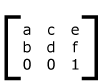Since only six values are used in the above 3x3 matrix, a transformation matrix is also expressed as a vector: [a b c d e f].

Transformations map coordinates and lengths from a new coordinate system into a previous coordinate system: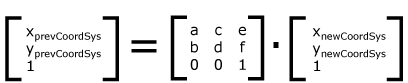Simple transformations are represented in matrix form as follows:

• Translation is equivalent to the matrix: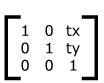or [1 0 0 1 tx ty], where tx and ty are the distances to translate coordinates in x and y, respectively.

• Scaling is equivalent to the matrix: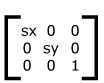or [sx 0 0 sy 0 0]. One unit in the x and y directions in the new coordinate system equals sx and sy units in the previous coordinate system, respectively.

• Rotation about the origin is equivalent to the matrix: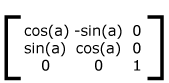or [cos(a) sin(a) -sin(a) cos(a) 0 0], which has the effect of rotating the coordinate system axes by angle a.

• A skew transformation along the x-axis is equivalent to the matrix: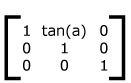or [1 0 tan(a) 1 0 0], which has the effect of skewing x coordinates by angle a.

• A skew transformation along the y-axis is equivalent to the matrix: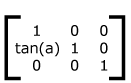or [1 tan(a) 0 1 0 0], which has the effect of skewing y coordinates by angle a.

## Nested transformations

Transformations can be nested to any level. The effect of nested transformations is to post-multiply (i.e., concatenate) the subsequent transformation matrices onto previously defined transformations: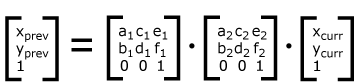For each given element, the accumulation of all transformations that have been defined on the given element and all of its ancestors up to and including the element that established the current viewport (usually, the 'svg' element which is the most immediate ancestor to the given element) is called the current transformation matrix or CTM. The CTM thus represents the mapping of current user coordinates to viewport coordinates: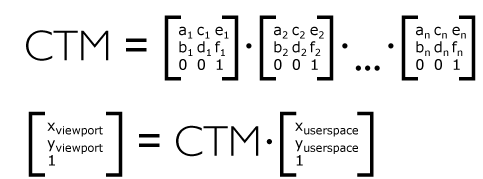Example 07_07 illustrates nested transformations.

In the example above, the CTM within the third nested transformation (i.e., the transform="translate(130,160)") consists of the concatenation of the three transformations, as follows: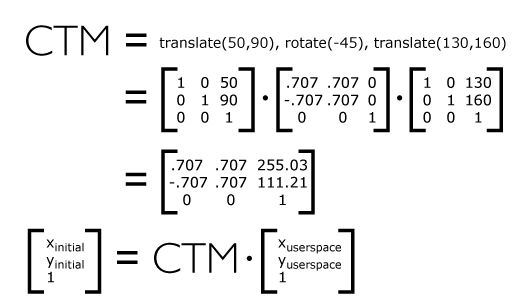## The 'transform' attribute

Attribute definition:

transform = "<transform-list>" | "<transform-ref>" | "none"

This attribute specifies a coordinate system transformation to apply to the element it appears on. The value of this attribute takes one of three forms:

<transform-list>

Specifies a list of affine transformations. See the definition in The TransformList value section below for details.

<transform-ref>

Specifies a constrained transformation. See the definition in The TransformRef value section below for details.

none

Specifies the identity transformation. Using this value has the same effect on the element's CTM as using the identity matrix (transform="matrix(1 0 0 1 0 0)") or not specifying the 'transform' attribute at all. This is the lacuna value.

Animatable: yes.

If the 'transform' attribute cannot be parsed according to the syntaxes above, then it has an unsupported value. In this case, as with any instance of an unsupported value, the SVG user agent must process the element as if the 'transform' attribute had not been specified, which will result in the element's transformation being the identity transformation.

### The TransformList value

A <transform-list> is defined as a list of transform definitions, which are applied in the order provided. The individual transform definitions are separated by white space and/or a comma. The available types of transform definitions are as follows:

• matrix(<a> <b> <c> <d> <e> <f>), which specifies a transformation in the form of a transformation matrix of six values. matrix(a,b,c,d,e,f) is equivalent to applying the transformation matrix [a b c d e f].

• translate(<tx> [<ty>]), which specifies a translation by tx and ty. If <ty> is not provided, it is assumed to be zero.

• scale(<sx> [<sy>]), which specifies a scale operation by sx and sy. If <sy> is not provided, it is assumed to be equal to <sx>.

• rotate(<rotate-angle> [<cx> <cy>]), which specifies a rotation by <rotate-angle> degrees about a given point.

If optional parameters <cx> and <cy> are not supplied, the rotation is about the origin of the current user coordinate system. The operation corresponds to the matrix [cos(a) sin(a) -sin(a) cos(a) 0 0].

If optional parameters <cx> and <cy> are supplied, the rotation is about the point (cxcy). The operation represents the equivalent of the following specification: translate(<cx>, <cy>) rotate(<rotate-angle>) translate(-<cx>, -<cy>).

• skewX(<skew-angle>), which specifies a skew transformation along the x-axis.

• skewY(<skew-angle>), which specifies a skew transformation along the y-axis.

All numeric values are real <number>s.

If the list of transforms includes a matrix with all values set to zero (that is, 'matrix(0,0,0,0,0,0)'), then rendering of the element is disabled. Such a value is not an unsupported value.

If a list of transforms includes more than one transform definition, then the net effect is as if each transform had been specified separately in the order provided. For example,

will have the same rendering as:

The 'transform' attribute is applied to an element before processing any other coordinate or length values supplied for that element. In the element

the 'x', 'y', 'width' and 'height', values are processed after the current coordinate system has been scaled uniformly by a factor of 2 by the 'transform' attribute. Attributes 'x', 'y', 'width' and 'height' (and any other attributes or properties) are treated as values in the new user coordinate system, not the previous user coordinate system. Thus, the above 'rect' element is functionally equivalent to:

The following is an EBNF grammar for <transform-list> values [EBNF]:

```transform-list ::=
wsp* transforms? wsp*
transforms ::=
transform
| transform comma-wsp+ transforms
transform ::=
matrix
| translate
| scale
| rotate
| skewX
| skewY
matrix ::=
"matrix" wsp* "(" wsp*
number comma-wsp
number comma-wsp
number comma-wsp
number comma-wsp
number comma-wsp
number wsp* ")"
translate ::=
"translate" wsp* "(" wsp* number ( comma-wsp number )? wsp* ")"
scale ::=
"scale" wsp* "(" wsp* number ( comma-wsp number )? wsp* ")"
rotate ::=
"rotate" wsp* "(" wsp* number ( comma-wsp number comma-wsp number )? wsp* ")"
skewX ::=
"skewX" wsp* "(" wsp* number wsp* ")"
skewY ::=
"skewY" wsp* "(" wsp* number wsp* ")"
number ::=
sign? integer-constant
| sign? floating-point-constant
comma-wsp ::=
(wsp+ comma? wsp*) | (comma wsp*)
comma ::=
","
integer-constant ::=
digit-sequence
floating-point-constant ::=
fractional-constant exponent?
| digit-sequence exponent
fractional-constant ::=
digit-sequence? "." digit-sequence
| digit-sequence "."
exponent ::=
( "e" | "E" ) sign? digit-sequence
sign ::=
"+" | "-"
digit-sequence ::=
digit
| digit digit-sequence
digit ::=
"0" | "1" | "2" | "3" | "4" | "5" | "6" | "7" | "8" | "9"
wsp ::=
(#x20 | #x9 | #xD | #xA)
```

## Constrained transformations

SVG 1.2 extends the coordinate system transformations allowed on container elements and graphics element to provide a method by which graphical objects can remain fixed in the viewport without being scaled or rotated. Use cases include thin lines that do not become fatter on zooming in, map symbols or icons of a constant size, and so forth.

The following summarizes the different transforms that are applied to a graphical object as it is rendered.

### The user transform

The user transform is the transformation that the SVG user agent positioning controls apply to the viewport coordinate system. This transform can be considered to be applied to a group that surrounds the 'svg' element of the document.

The SVG user agent positioning controls consist of a translation (commonly referred to as the "pan"), a scale (commonly referred to as the "zoom") and a rotate.

```US = Matrix corresponding to the user scale  (currentScale on SVGSVGElement)
UP = Matrix corresponding to the user pan    (currentTranslate on SVGSVGElement)
UR = Matrix corresponding to the user rotate (currentRotate on SVGSVGElement)```

The user transform is the product of these component transformations.

```U = User transform
= UP . US . UR```

### ViewBox to viewport transformation

Some SVG elements, such as the rootmost 'svg' element, create their own viewport. The 'viewBox' to viewport transformation is the transformation on an 'svg' element that adjusts the coordinate system to take the 'viewBox' and 'preserveAspectRatio' attributes into account.

We use the following notation for a 'viewBox' to viewport transformation:

`VB(svgId)`

The svgId parameter is the value of the 'id' or 'xml:id' attribute on a given 'svg' element.

### Element transform stack

All elements in an SVG document have a transform stack. This is the list of transforms that manipulate the coordinate system between the element and its nearest ancestor 'svg' element, i.e. in this specification, the root element.

We use the following notation for the element transform stack on a given element:

`TS(id)`

The id parameter is the value of the 'id' or 'xml:id' attribute on a given element.

Similarly, we use the following notation for the transform defined by the 'transform' attribute on the given element with identifier id:

`Txf(id)`

With the above definition, the transformation TS of an element is equal to the product of all the transformations Txf from that element to its nearest ancestor 'svg'.

`TS(id) = Txf(id.nearestViewportElement) . [...] . Txf(id.parentElement) . Txf(id)`
Example: Element transform stack
```

]]>```

In this example, the transforms are:

```TS(g)  = scale(2)
TS(r)  = TS(g) . scale(4)   = scale(8)
TS(g2) = TS(g) . I          = scale(2)   (where I is the identity matrix)
TS(r2) = TS(g) . scale(0.5) = scale(1)
```

### The current transformation matrix

Each element in the rendering tree has the concept of a current transformation matrix or CTM. This is the product of all coordinate system transformations that apply to an element, effectively mapping the element into a coordinate system that is then transformed into device units by the SVG user agent.

Consider the following example, with a rectangle having a set of ancestor 'g' elements with IDs "g-0" to "g-n".

Example: Current transformation matrix
```
...

...

...

...

...

]]>```

With the above definitions for U, VB, and TS, the CTM for the rectangle with xml:id="elt" is computed as follows:

```CTM(elt) = U . VB(root) . TS(elt)
= U . VB(root) . Txf(g-n) . [...] . Txf(g-0) . Txf(elt)
```
Example: Current transformation matrix, n=2
```
...

...

...

]]>```

This produces the following transformations:

```CTM(elt) = U . VB(root) . Txf(g-1) . Txf(g-0) . Txf(elt)
```

Note the important relationship between an element's CTM and its parent CTM, for elements which do not define a viewport:

`CTM(elt) = CTM(elt.parentElement) . Txf(elt)`

### The TransformRef value

By using the 'ref(...)' attribute value on the 'transform' attribute it is possible to specify simple constrained transformations.

The 'ref(svg, x, y)' transform evaluates to the inverse of the element's parent's CTM multiplied by the rootmost 'svg' element's CTM but exclusive of that 'svg' element's zoom/pan/rotate user transform, if any.

Note that the inverse of the parent element's CTM may not always exist. In such cases, the user agent can instead calculate the CTM of the element with the constrained transformation by looking up the CTM of the rootmost 'svg' element directly. The 'ref(...)' value in this case is not an unsupported value.

The x and y parameters are optional. If they are specified, an additional translation is appended to the transform so that (0, 0) in the element's user space maps to (xy) in the 'svg' element's user space. If no x and y parameters are specified, no additional translation is applied.

Using the definitions provided above, and using "svg" to denote the rootmost 'svg' element:

```Inverse of the parent's CTM: inv(CTM(elt.parentElement))

The svg element's user transform, exclusive of zoom,
pan and rotate transforms:
CTM(svg.parentElement) . VB(svg)

CTM(svg.parentElement) evaluates to Identity since there
is no svg.parentElement element.```

In addition, the T(x, y) translation is such that:

`CTM(elt) . (0, 0) = CTM(svg) . (x, y)`

So the transform evaluates to:

`Txf(elt) = inv(CTM(elt.parentElement)) . CTM(svg.parentElement) . VB(svg) . T(x, y)`

Thus, the element's CTM is:

```CTM(elt) = CTM(elt.parentElement) . Txf(elt)
= CTM(svg.parentElement) . VB(svg) . T(x,y)```
Example: ref() transform
A small rectangle initially marks the middle of a line. The SVG user agent viewport is a square with sides of 200 units.
```

]]>```

In this case:

```Txf(r) = inv(CTM(r.parent)) . CTM(root.parentElement) . VB(root) . T(x, y)

CTM(root.parentElement) evaluates to Identity.

T(x, y) evaluates to Identity because (x, y) is not specified

CTM(r) = CTM(r.parent) . Txf(r)
= CTM(r.parent) . inv(CTM(r.parent)) . VB(root)
= VB(root)
= scale(2)
```

Consequently, regardless of the user transform (due to `currentTranslate`, `currentScale` and `currentRotate`) the rectangle's coordinates in viewport space will always be: (45, 45, 10, 10) * scale(2) = (90, 90, 20, 20). Initially, the line is from (0, 0) to (200, 200) in the viewport coordinate system. If we apply a user agent zoom of 3 (`currentScale` = 3), the rectangle is still (90, 90, 20, 20) but the line is (0, 0, 600, 600) and the marker no longer marks the middle of the line.

Example: ref() transform
A small rectangle always marks the middle of a line. Again, the SVG user agent viewport is a square with sides of 200 units.
```

]]>```

In this case:

```Txf(g) = inv(CTM(g.parent)) . CTM(root.parentElement) . VB(root) . T(x,y)

CTM(root.parentElement) evaluates to Identity.

CTM(g) = CTM(g.parent) . Txf(r)
= CTM(g.parent) . inv(CTM(g.parent)) . VB(root) . T(x,y)
= VB(root) . T(x,y)
= scale(2) . T(x,y)
```

Initially, (50, 50) in the 'svg' user space is (100, 100) in viewport space. Therefore:

```CTM(g) . [0, 0] = CTM(root) . [50, 50]
= scale(2) . [50, 50]
= [100, 100]

and

scale(2) . T(x,y) = [100, 100]

T(x,y) = translate(50, 50)
```

If the SVG user agent pan was (50, 80) (modifying `currentTranslate`) then we now have (50, 50) in the 'svg' element's user space located at (150, 180) in viewport space. This produces:

```CTM(g) . [0, 0] = CTM(root) . [50, 50]
= translate(50, 80) . scale(2) . [50, 50]
= [150, 180]

and

scale(2) . T(x,y) = [150, 180]

T(x, y) = translate(75, 90)
```

Therefore, regardless of the user transform, the rectangle will always overlap the middle of the line. Note that the rectangle will not rotate with the line (e.g., if `currentRotate` is set) and it will not scale either.

The following is an EBNF grammar for <transform-ref> values [EBNF]:

```transform-ref ::=
wsp* ref wsp*
ref ::=
"ref" wsp* "(" wsp* "svg" wsp* ")"
| "ref" wsp* "(" wsp* "svg" comma-wsp number comma-wsp number wsp* ")"
number ::=
sign? integer-constant
| sign? floating-point-constant
comma-wsp ::=
(wsp+ comma? wsp*) | (comma wsp*)
comma ::=
","
integer-constant ::=
digit-sequence
floating-point-constant ::=
fractional-constant exponent?
| digit-sequence exponent
fractional-constant ::=
digit-sequence? "." digit-sequence
| digit-sequence "."
exponent ::=
( "e" | "E" ) sign? digit-sequence
sign ::=
"+" | "-"
digit-sequence ::=
digit
| digit digit-sequence
digit ::=
"0" | "1" | "2" | "3" | "4" | "5" | "6" | "7" | "8" | "9"
wsp ::=
(#x20 | #x9 | #xD | #xA)
```

## The 'viewBox' attribute

It is often desirable to specify that a given set of graphics stretch to fit a particular container element. The 'viewBox' attribute provides this capability. All elements that establish a new viewport (see elements that establish viewports) can have the 'viewBox' attribute specified on them.

Attribute definition:

viewBox = "<list-of-numbers>" | "none"

Specifies a rectangular region into which child graphical content must be fit. The value of this attribute takes one of two forms:

<list-of-numbers>

A list of four <number>s (<min-x>, <min-y>, <width> and <height>), separated by white space and/or a comma, which specify a rectangle in viewport space which must be mapped to the bounds of the viewport established by the given element, taking into account the 'preserveAspectRatio' attribute. If specified, an additional transformation is applied to all descendants of the given element to achieve the specified effect.

none

Specifying a value of "none" indicates that a supplemental transformation due to the 'viewBox' attribute must not be used. Using this value will have the same affect on child content as not specifying the 'viewBox' attribute at all. This is the lacuna value.

Animatable: yes.

A negative value for <width> or <height> is unsupported. A value of zero for either of these two parameters disables rendering of the element.

Example 07_12 illustrates the use of the 'viewBox' attribute on the 'svg' element to specify that the SVG content must stretch to fit the bounds of the viewport.

Rendered into viewport with width=300px, height=200px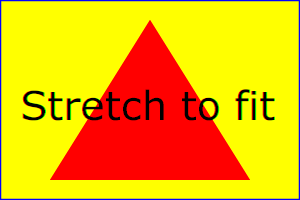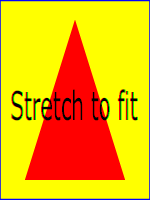The effect of the 'viewBox' attribute is that the SVG user agent automatically supplies the appropriate transformation matrix to map the specified rectangle in user space to the bounds of a designated region (often, the viewport). To achieve the effect of the example on the left, with viewport dimensions of 300 by 200 pixels, the SVG user agent needs to automatically insert a transformation which scales both x and y by 0.2. The effect is equivalent to having a viewport of size 300px by 200px and the following supplemental transformation in the document, as follows:

```<svg xmlns="http://www.w3.org/2000/svg" version="1.2" baseProfile="tiny"
width="300px" height="200px">
<g transform="scale(0.2)">
<!-- Rest of document goes here -->
</g>
</svg>
```

To achieve the effect of the example on the right, with viewport dimensions of 150 by 200 pixels, the SVG user agent needs to automatically insert a transformation which scales x by 0.1 and y by 0.2. The effect is equivalent to having a viewport of size 150px by 200px and the following supplemental transformation in the document, as follows:

```<svg xmlns="http://www.w3.org/2000/svg" version="1.2" baseProfile="tiny"
width="150px" height="200px">
<g transform="scale(0.1 0.2)">
<!-- Rest of document goes here -->
</g>
</svg>
```

(Note: in some cases the SVG user agent will need to supply a translate transformation in addition to a scale transformation. For example, on an 'svg' element, a translate transformation will be needed if the 'viewBox' attribute specifies values other than zero for <min-x> or <min-y>.)

Unlike the 'transform' attribute (see effect of the 'transform' attribute on sibling attributes), the automatic transformation that is created due to a 'viewBox' does not affect the 'x', 'y', 'width' and 'height' attributes on the element with the 'viewBox' attribute. Thus, in the example above which shows an 'svg' element which has attributes 'width', 'height' and 'viewBox', the 'width' and 'height' attributes represent values in the coordinate system that exists before the 'viewBox' transformation is applied. On the other hand, like the 'transform' attribute, it does establish a new coordinate system for all other attributes and for descendant elements.

The following is an EBNF grammar for values of the 'viewBox' attribute [EBNF]:

```viewbox ::=
wsp* viewboxSpec wsp*
viewboxSpec ::=
number comma-wsp number comma-wsp number comma-wsp number
| "none"
number ::=
sign? integer-constant
| sign? floating-point-constant
comma-wsp ::=
(wsp+ comma? wsp*) | (comma wsp*)
comma ::=
","
integer-constant ::=
digit-sequence
floating-point-constant ::=
fractional-constant exponent?
| digit-sequence exponent
fractional-constant ::=
digit-sequence? "." digit-sequence
| digit-sequence "."
exponent ::=
( "e" | "E" ) sign? digit-sequence
sign ::=
"+" | "-"
digit-sequence ::=
digit
| digit digit-sequence
digit ::=
"0" | "1" | "2" | "3" | "4" | "5" | "6" | "7" | "8" | "9"
wsp ::=
(#x20 | #x9 | #xD | #xA)
```

## The 'preserveAspectRatio' attribute

In some cases, typically when using the 'viewBox' attribute, it is desirable that the graphics stretch to fit non-uniformly to take up the entire viewport. In other cases, it is desirable that uniform scaling be used for the purposes of preserving the aspect ratio of the graphics.

'preserveAspectRatio' is available for all elements that establish a new viewport (see elements that establish viewports), indicates whether or not to force uniform scaling.

'preserveAspectRatio' only applies when a value has been provided for 'viewBox' on the same element. Or, in some cases, if an implicit 'viewBox' value can be established for the element (see each element description for details on this). If a 'viewBox' value can not be determined then 'preserveAspectRatio' is ignored.

Attribute definition:

preserveAspectRatio = ["defer"] <align> [<meet>]
defer
If the value of 'preserveAspectRatio' on an element that references data ('image', 'animation' and 'video') starts with defer then the value of the 'preserveAspectRatio' attribute on the referenced content if present must be used.  If the referenced content lacks a value for 'preserveAspectRatio' then the 'preserveAspectRatio' attribute must be processed as normal (ignoring defer).  For 'preserveAspectRatio' on all other elements the defer portion of the attribute is ignored.
<align>
Indicates whether to force uniform scaling and, if so, the alignment method to use in case the aspect ratio of the 'viewBox' doesn't match the aspect ratio of the viewport. The <align> parameter must be one of the following strings:
• none - Do not force uniform scaling. Scale the graphic content of the given element non-uniformly if necessary such that the element's bounding box exactly matches the viewport rectangle.
• xMinYMin - Force uniform scaling.
Align the <min-x> of the element's 'viewBox' with the smallest X value of the viewport.
Align the <min-y> of the element's 'viewBox' with the smallest Y value of the viewport.
• xMidYMin - Force uniform scaling.
Align the midpoint X value of the element's 'viewBox' with the midpoint X value of the viewport.
Align the <min-y> of the element's 'viewBox' with the smallest Y value of the viewport.
• xMaxYMin - Force uniform scaling.
Align the <min-x>+<width> of the element's 'viewBox' with the maximum X value of the viewport.
Align the <min-y> of the element's 'viewBox' with the smallest Y value of the viewport.
• xMinYMid - Force uniform scaling.
Align the <min-x> of the element's 'viewBox' with the smallest X value of the viewport.
Align the midpoint Y value of the element's 'viewBox' with the midpoint Y value of the viewport.
• xMidYMid (the lacuna value) - Force uniform scaling.
Align the midpoint X value of the element's 'viewBox' with the midpoint X value of the viewport.
Align the midpoint Y value of the element's 'viewBox' with the midpoint Y value of the viewport.
• xMaxYMid - Force uniform scaling.
Align the <min-x>+<width> of the element's 'viewBox' with the maximum X value of the viewport.
Align the midpoint Y value of the element's 'viewBox' with the midpoint Y value of the viewport.
• xMinYMax - Force uniform scaling.
Align the <min-x> of the element's 'viewBox' with the smallest X value of the viewport.
Align the <min-y>+<height> of the element's 'viewBox' with the maximum Y value of the viewport.
• xMidYMax - Force uniform scaling.
Align the midpoint X value of the element's 'viewBox' with the midpoint X value of the viewport.
Align the <min-y>+<height> of the element's 'viewBox' with the maximum Y value of the viewport.
• xMaxYMax - Force uniform scaling.
Align the <min-x>+<width> of the element's 'viewBox' with the maximum X value of the viewport.
Align the <min-y>+<height> of the element's 'viewBox' with the maximum Y value of the viewport.
<meet>
Optional and only available due to historical reasons. The <meet> is separated from the <align> value by one or more spaces and must equal the string meet.

meet indicates to scale the graphic such that:
• aspect ratio is preserved
• the entire 'viewBox' is visible within the viewport
• the 'viewBox' is scaled up as much as possible, while still meeting the other criteria
In this case, if the aspect ratio of the graphic does not match the viewport, some of the viewport will extend beyond the bounds of the 'viewBox' (i.e., the area into which the 'viewBox' will draw will be smaller than the viewport).

Animatable: yes.

Example PreserveAspectRatio illustrates the various options on 'preserveAspectRatio'. The example creates several new viewports by including 'animation' elements (see Establishing a new viewport).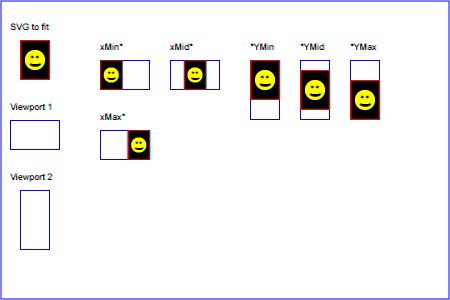## Establishing a new viewport

Some elements establish a new viewport. By establishing a new viewport, you implicitly establish a new viewport coordinate system and a new user coordinate system. Additionally, there is a new meaning for percentage units defined to be relative to the current viewport since a new viewport has been established (see Units).

'viewport-fill' and 'viewport-fill-opacity' properties can be applied on the new viewport.

The bounds of the new viewport are defined by the 'x', 'y', 'width' and 'height' attributes on the element establishing the new viewport, such as an 'animation' element. Both the new viewport coordinate system and the new user coordinate system have their origins at (xy), where x and y represent the value of the corresponding attributes on the element establishing the viewport. The orientation of the new viewport coordinate system and the new user coordinate system correspond to the orientation of the current user coordinate system for the element establishing the viewport. A single unit in the new viewport coordinate system and the new user coordinate system are the same size as a single unit in the current user coordinate system for the element establishing the viewport.

For an extensive example of creating new viewports, see Example PreserveAspectRatio.

The following elements establish new viewports:

The following paragraph is informative.

Note that no clipping of overflow is performed, but that such clipping will take place if the content is viewed in an SVG user agent that supports clipping (e.g. a user agent that supports SVG 1.1 Full [SVG11]), since the initial value for the 'overflow' property is hidden for non-root elements that establish viewports ([SVG11], section 14.3.3). Content authors that want content to be fully forward compatible are advised to either specify the 'overflow' property or to make sure that content that shouldn't be clipped is inside of the established viewport.

## Units

Besides the exceptions listed below all coordinates and lengths in SVG must be specified in user units, which means that unit identifiers are not allowed.
Two exceptions exist:

A user unit is a value in the current user coordinate system. For example:

For the 'svg' element's 'width' and 'height' attributes a coordinate or length value can be expressed as a number following by a unit identifier (e.g., '25cm' or '100%'). The list of unit identifiers in SVG are: in, cm, mm, pt, pc, px and percentages (%). These values on 'width' and 'height' contribute towards the calculation of the initial viewport.

Using percentage values on 'width' and 'height' attributes mandates how much space the SVG viewport must take of the available initial viewport. In particular:

See the discussion on the initial viewport for more details.

## Bounding box

The bounding box (or "bbox") of an element is the tightest fitting rectangle aligned with the axes of that element's user coordinate system that entirely encloses it and its descendants. The bounding box must be computed exclusive of any values for the fill related properties, the stroke related properties, the opacity related properties or the visibility property. For curved shapes, the bounding box must enclose all portions of the shape along the edge, not just end points. Note that control points for a curve which are not defined as lying along the line of the resulting curve (e.g., the second coordinate pair of a Cubic Bézier command) must not contribute to the dimensions of the bounding box (though those points may fall within the area of the bounding box, if they lie within the shape itself, or along or close to the curve). For example, control points of a curve that are at a further distance than the curve edge, from the non-enclosing side of the curve edge, must be excluded from the bounding box.

Example bbox01 shows one shape (a 'path' element with a quadratic Bézier curve) with three possible bounding boxes, only the leftmost of which is correct.

The bounding box must be applicable for any rendering element with positive 'width' or 'height' attributes and with a 'display' property other than none, as well as for any container element that may contain such elements. Elements which do not partake in the rendering tree (e.g. elements in a 'defs' element, elements whose 'display' is none, etc.), and which have no child elements that partake in the rendering tree (e.g. 'g' elements with no children), shall not contribute to the bounding box of the parent element. Elements that do not contribute to the bounding box of a parent element must still return their own bounding box value when required.

To illustrate, example bbox-calc below shows a set of elements. Given this example, the following results shall be calculated for each of the elements.

Element ID Bounding Box Result
"`defs-1`" {0, 0, 0, 0}
"`rect-1`" {20, 20, 40, 40}
"`group-1`" {30, 30, 40, 40}
"`use-1`" {30, 30, 40, 40}
"`group-2`" {10, 10, 100, 100}
"`rect-2`" {10, 10, 100, 100}

Elements and document fragments which derive from SVGLocatable but are not in the rendering tree, such as those in a 'defs' element or those which have been been created but not yet inserted into the DOM, must still have a bounding box. The geometry of elements outside the rendering tree must take into account only those properties and values (such as 'font-size') which are specified within that element or document fragment, or which have a lacuna value or an implementation-defined value.

For text content elements, for the purposes of the bounding box calculation, each glyph must be treated as a separate graphics element. The calculations must assume that all glyphs occupy the full glyph cell. For example, for horizontal text, the calculations must assume that each glyph extends vertically to the full ascent and descent values for the font. An exception to this is the 'textArea', which uses that element's geometry for the bounding box calculation.

Because declarative or scripted animation can change the shape, size, and position of an element, the bounding box is mutable. Thus, the bounding box for an element shall reflect the current values for the element at the snapshot in time at which the bounding box is requested, whether through a script call or as part of a declarative or linking syntax.

Note that an element which has either or both of 'width' and 'height' of '0' (such as a vertical or horizontal line, or a 'rect' element with an unspecified 'width' or 'height') still has a bounding box, with a positive value for the positive dimension, or with '0' for both 'width' and 'height' if no positive dimension is specified. Similarly, subpaths segments of a 'path' element with '0' 'width' and 'height' must be included in that element's geometry for the sake of the bounding box. Note also that elements which do not derive from SVGLocatable (such as gradient elements) do not have a bounding box, thus have no interface to request a bounding box.

Elements in the rendering tree which reference unresolved resources shall still have a bounding box, defined by the position and dimensions specified in their attributes, or by the lacuna value for those attributes if no values are supplied. For example, the element `<use xlink:href="#bad" x="10" y="10"/>` would have a bounding box with an 'x' and 'y' of '10' and a 'width' and 'height' of '0'.

For a formal definition of bounding boxes, see [FOLEY-VANDAM], section 15.2.3, Extents and Bounding Volumes. For further details, see bounding box calculations, the effects of visibility on bounding box, object bounding box units and text elements, and fragment identifiers.

## Object bounding box units

The following elements offer the option of expressing coordinate values and lengths as fractions of the bounding box (via keyword 'objectBoundingBox') on a given element:

Element Attribute Effect
'linearGradient' gradientUnits="objectBoundingBox" Indicates that the attributes which specify the gradient vector ('x1', 'y1', 'x2', 'y2') represent fractions of the bounding box of the element to which the gradient is applied.
'radialGradient' gradientUnits="objectBoundingBox" Indicates that the attributes which specify the center ('cx', 'cy') and the radius ('r') represent fractions of the bounding box of the element to which the gradient is applied.

In the discussion that follows, the term applicable element is the element to which the given effect applies. For gradients the applicable element is the graphics element which has its 'fill' or 'stroke' property referencing the given gradient. (See Inheritance of painting properties. For special rules concerning text elements, see the discussion of object bounding box units and text elements.)

When keyword 'objectBoundingBox' is used, then the effect is as if a supplemental transformation matrix were inserted into the list of nested transformation matrices to create a new user coordinate system.

First, the (minxminy) and (maxxmaxy) coordinates are determined for the applicable element and all of its descendants. The values minx, miny, maxx and maxy are determined by computing the maximum extent of the shape of the element in x and y with respect to the user coordinate system for the applicable element.

Then, coordinate (0, 0) in the new user coordinate system is mapped to the (minxminy) corner of the tight bounding box within the user coordinate system of the applicable element and coordinate (1, 1) in the new user coordinate system is mapped to the (maxxmaxy) corner of the tight bounding box of the applicable element. In most situations, the following transformation matrix produces the correct effect:

```[ (maxx-minx) 0 0 (maxy-miny) minx miny ]
```

Any numeric value can be specified for values expressed as a fraction of object bounding box units. In particular, fractions less are zero or greater than one can be specified.

Keyword 'objectBoundingBox' should not be used when the geometry of the applicable element has no width or no height, such as the case of a horizontal or vertical line, even when the line has actual thickness when viewed due to having a non-zero stroke width since stroke width is ignored for bounding box calculations. When the geometry of the applicable element has no width or height and 'objectBoundingBox' is specified, then the given effect (e.g., a gradient) will be ignored.

## Intrinsic sizing properties of the viewport of SVG content

SVG needs to specify how to calculate some intrinsic sizing properties to enable inclusion within other languages. The intrinsic width and height of the viewport of SVG content must be determined from the 'width' and 'height' attributes. If either of these are not specified, the lacuna value of '100%' must be used. Note: the 'width' and 'height' attributes are not the same as the CSS width and height properties. Specifically, percentage values do not provide an intrinsic width or height, and do not indicate a percentage of the containing block. Rather, they indicate the portion of the viewport that is actually covered by image data.

The intrinsic aspect ratio of the viewport of SVG content is necessary for example, when including SVG from an object element in XHTML styled with CSS. It is possible (indeed, common) for an SVG graphic to have an intrinsic aspect ratio but not to have an intrinsic width or height. The intrinsic aspect ratio must be calculated based upon the following rules:

Examples:

Example: Intrinsic Aspect Ratio 1
```
...
]]>```

In this example the intrinsic aspect ratio of the viewport is 2:1. The intrinsic width is 10cm and the intrinsic height is 5cm.

Example: Intrinsic Aspect Ratio 2
```
...
]]>```

In this example the intrinsic aspect ratio of the rootmost viewport is 1:1. An aspect ratio calculation in this case allows embedding in an object within a containing block that is only constrained in one direction.

Example: Intrinsic Aspect Ratio 3
```
...
]]>```

In this case the intrinsic aspect ratio is 1:1.

Example: Intrinsic Aspect Ratio 4
```
...
]]>```

In this example, the intrinsic aspect ratio is 1:1.

## Geographic coordinate systems

In order to allow interoperability between SVG content generators and SVG user agents dealing with maps encoded in SVG, the use of a common metadata definition for describing the coordinate system used to generate SVG documents is encouraged.

Such metadata must be added under the 'metadata' element of the topmost 'svg' element describing the map, consisting of an RDF description of the Coordinate Reference System definition used to generate the SVG map [RDF]. Note that the presence of this metadata does not affect the rendering of the SVG in any way; it merely provides added semantic value for applications that make use of combined maps.

The definition must be conformant to the XML grammar described in GML 3.2.1, an OpenGIS Standard for encoding common CRS data types in XML [GML]. In order to correctly map the 2-dimensional data used by SVG, the CRS must be of subtype ProjectedCRS or Geographic2dCRS. The first axis of the described CRS maps the SVG x-axis and the second axis maps the SVG y-axis.

The main purpose of such metadata is to indicate to the user agent that two or more SVG documents can be overlayed or merged into a single document. Obviously, if two maps reference the same Coordinate Reference System definition and have the same SVG 'transform' attribute value then they can be overlayed without reprojecting the data. If the maps reference different Coordinate Reference Systems and/or have different SVG 'transform' attribute values, then a specialized cartographic user agent may choose to transform the coordinate data to overlay the data. However, typical SVG user agents are not required to perform these types of transformations, or even recognize the metadata. It is described in this specification so that the connection between geographic coordinate systems and the SVG coordinate system is clear.

## The 'svg:transform' attribute

Attribute definition:

svg:transform = "<transform>" | "none"
<transform>

Specifies the affine transformation that has been applied to the map data. The syntax is identical to that described in The 'transform' attribute section.

none

Specifies that no supplemental affine transformation has been applied to the map data. Using this value has the same meaning as specifying the identity matrix, which in turn is just the same as not specifying the 'svg:transform' the attribute at all.

Animatable: no.

This attribute describes an optional additional affine transformation that may have been applied during this mapping. This attribute may be added to the OpenGIS 'CoordinateReferenceSystem' element. Note that, unlike the 'transform' attribute, it does not indicate that a transformation is to be applied to the data within the file. Instead, it simply describes the transformation that was already applied to the data when being encoded in SVG.

There are three typical uses for the 'svg:transform' global attribute. These are described below and used in the examples.

• Most ProjectedCRS have the north direction represented by positive values of the second axis and conversely SVG has a y-down coordinate system. That's why, in order to follow the usual way to represent a map with the north at its top, it is recommended for that kind of ProjectedCRS to use the 'svg:transform' global attribute with a 'scale(1, -1)' value as in the third example below.

• Most Geographic2dCRS have the latitude as their first axis rather than the longitude, which means that the south-north axis would be represented by the x-axis in SVG instead of the usual y-axis. That's why, in order to follow the usual way to represent a map with the north at its top, it is recommended for that kind of Geographic2dCRS to use the 'svg:transform' global attribute with a 'rotate(-90)' value as in the first example (while also adding the 'scale(1, -1)' as for ProjectedCRS).

• In addition, when converting for profiles which place restrictions on precision of real number values, it may be useful to add an additional scaling factor to retain good precision for a specific area. When generating an SVG document from WGS84 geographic coordinates (EPGS 4326), we recommend the use of an additional 100 times scaling factor corresponding to an 'svg:transform' global attribute with a 'rotate(-90) scale(100)' value (shown in the second example). Different scaling values may be required depending on the particular CRS.

Below is a simple example of the coordinate metadata, which describes the coordinate system used by the document via a URI.

The second example uses a well-known identifier to describe the coordinate system. Note that the coordinates used in the document have had the supplied transform applied.

The third example defines the coordinate system completely within the SVG document.